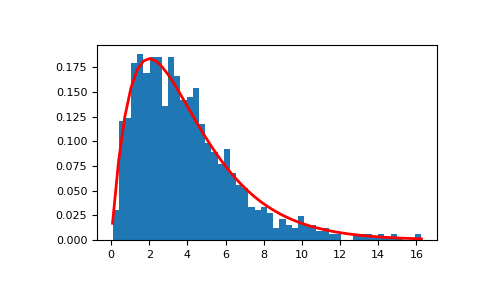numpy.random.mtrand.RandomState.gamma¶

method

RandomState.gamma(shape, scale=1.0, size=None)

Draw samples from a Gamma distribution.

Samples are drawn from a Gamma distribution with specified parameters, shape (sometimes designated “k”) and scale (sometimes designated “theta”), where both parameters are > 0.

Parameters: shape : float or array_like of floats The shape of the gamma distribution. Must be non-negative. scale : float or array_like of floats, optional The scale of the gamma distribution. Must be non-negative. Default is equal to 1. size : int or tuple of ints, optional Output shape. If the given shape is, e.g., (m, n, k), then m * n * k samples are drawn. If size is None (default), a single value is returned if shape and scale are both scalars. Otherwise, np.broadcast(shape, scale).size samples are drawn. out : ndarray or scalar Drawn samples from the parameterized gamma distribution.

scipy.stats.gamma
probability density function, distribution or cumulative density function, etc.

Notes

The probability density for the Gamma distribution iswhereis the shape andthe scale, andis the Gamma function.

The Gamma distribution is often used to model the times to failure of electronic components, and arises naturally in processes for which the waiting times between Poisson distributed events are relevant.

References

  Weisstein, Eric W. “Gamma Distribution.” From MathWorld–A Wolfram Web Resource. http://mathworld.wolfram.com/GammaDistribution.html
  Wikipedia, “Gamma distribution”, https://en.wikipedia.org/wiki/Gamma_distribution

Examples

Draw samples from the distribution:

>>> shape, scale = 2., 2.  # mean=4, std=2*sqrt(2)
>>> s = np.random.gamma(shape, scale, 1000)

Display the histogram of the samples, along with the probability density function:

>>> import matplotlib.pyplot as plt
>>> import scipy.special as sps  # doctest: +SKIP
>>> count, bins, ignored = plt.hist(s, 50, density=True)
>>> y = bins**(shape-1)*(np.exp(-bins/scale) /  # doctest: +SKIP
...                      (sps.gamma(shape)*scale**shape))
>>> plt.plot(bins, y, linewidth=2, color='r')  # doctest: +SKIP
>>> plt.show()Previous topic

numpy.random.mtrand.RandomState.f

Next topic

numpy.random.mtrand.RandomState.geometric